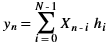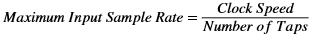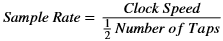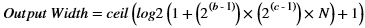# Single Multiplier MACC FIR Filter

## Versal ACAP DSP Engine Architecture Manual (AM004)

Document ID
AM004
Release Date
2022-09-11
Revision
1.2.1 English

The single-multiplier MACC FIR is one of the simplest DSP filter structures. The MACC structure uses a single multiplier with an accumulator to implement a FIR filter sequentially versus a full parallel FIR filter. This trade-off not only reduces hardware by a factor of N, but also reduces filter throughput by the same factor. The general FIR filter equation is a summation of products (also known as an inner product), defined as follows.In this equation, a set of N coefficients is multiplied by N respective data samples, and the inner products are summed together to form an individual result. The values of the coefficients determine the characteristics of the filter (for example, low-pass filter, band-pass filter, and high-pass filter). The equation can be mapped to many different implementations (for example, sequential, semi-parallel, or parallel) in the different available architectures.

Refer to the Resource Utilization Guideline for an implementation of the MACC FIR filter and the guideline on choosing the resource to implement the data buffer. In the example design, the implementation uses SRL16 for the data buffer. To support rounding toward infinity, apply the value of (2^(fractional_part-1)) -1 to the C input. The multiplier followed by the accumulator sums the products over the same number of cycles as there are coefficients. With this relationship, the performance of the MACC FIR filter is calculated using the following equation.If the coefficients possess a symmetric shape, a slightly costlier structure is available (see Symmetric MACC FIR Filter), however, the maximum sampled rate is doubled. The sample rate of the costlier structure is defined as follows.The nature of the FIR filter, with numerous MACC operations, outputs a larger number of bits from the filter than are present on the filters input. This effect is the bit growth or the gain of a filter. Due to the large output width, the full precision result is typically rounded and quantized. However, it is important to calculate the full precision output to select the correct bits from the output of the MACC.

One technique assumes every value in the filter could be the worst possible for the size of the two's complement numbers specified. Using the generic saturation level is a good starting point when the coefficients are unknown, but the number of bits required to represent them is known, for example, if the coefficients are re-loadable, as in adaptive filters. The equation of the output width in this case is given as follows.where:
• ceil: Rounds up to the nearest integer
• b: Number of bits in the data samples
• c: Number of bits in the coefficients

It is possible to use two clocks in the MACC FIR implementation. The faster clock goes to the DSP and the coefficient memory; the slow clock goes to the PL. It is therefore possible to avoid the condition in which the input has to be held for the number of taps cycles (the input throughput in the implementation using one clock only is equal to the number of taps).

The reference design files associated with the two MACC FIR Filter use cases are available in the macc_FIR/macc_FIR and macc_FIR/macc_FIR_2_clocks directories in the design archive file, am004-versal-dsp-engine.zip.